Next: Formulas. Up: Quiz3 Previous: Guidelines.

#### Notations.

The set of non-negative integer numbers, integer numbers and rational numbers are denoted respectively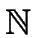,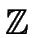and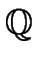, as usual. For an integer n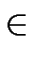the set of the multiples of n (all of them: positive, null or negative) is denoted by n. For an integer nthe residue class ring of the binary relation (a, b)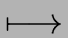a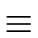b modn is denoted by/n, as usual. For a ring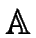, we denote by[x] the ring of univariate polynomials in x with coefficients in.Next: Formulas. Up: Quiz3 Previous: Guidelines.
Marc Moreno Maza
2006-01-09